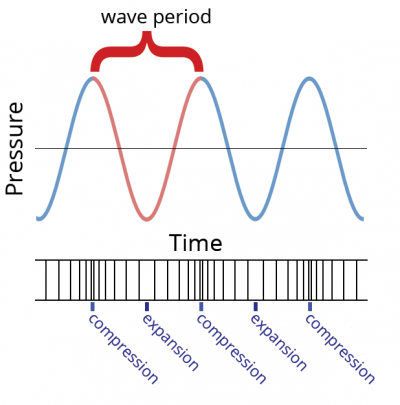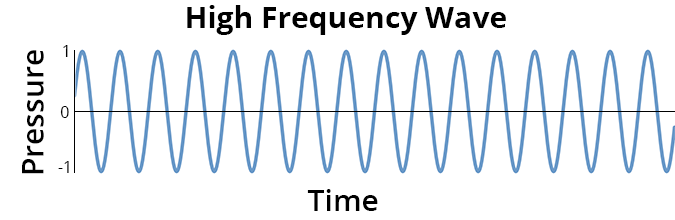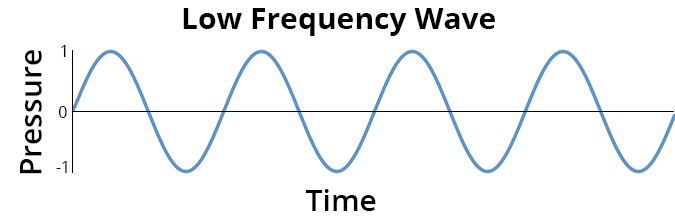# Frequency

High-pitched or low-pitched refer to the frequency of a sound wave. Because sound travels in a wave, the characteristics that scientists use to describe sound, such as frequency, can be related to a picture of a wave.  A wave has a repeating pattern. One complete repetition is called a cycle. The time to complete a cycle is the period. Frequency is the number of cycles per second.  The unit used to measure frequency is named hertz, which is defined to be the number of cycles in one second. (The hertz unit is named after Heinrich Hertz, a famous 19th century physicist.)The following video shows a high frequency wave and a low frequency wave, plotted as pressure versus time. There are 15 cycles in the high frequency wave and only 3 cycles in the low frequency wave, for the same time duration.If you increase the frequency of sound (there are more cycles in a second), you get a higher pitched sound. When you decrease the frequency, you get a lower pitched sound.

DOSITS Frequency short video.

Listen to the difference between frequencies in the series of sounds below.

A series of tones from 200Hz to 475Hz at 25Hz intervals.